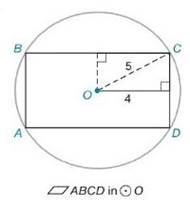Chapter 8.2, Problem 4EElementary Geometry For College St...

7th Edition
Alexander + 2 others
ISBN: 9781337614085

Solutions

Chapter
SectionElementary Geometry For College St...

7th Edition
Alexander + 2 others
ISBN: 9781337614085
Textbook Problem

In Exercises 1 to 8, find the perimeter of each polygonTo determine

To Find:

Perimeter of the rectangle provided.

Explanation

Formula Used:

The perimeter of a polygon is the sum of the lengths of all sides of the polygon.

Pythagorean theorem for the right angle triangle ABC for the hypotenuse AC,

AC2=AB2+BC2.

Perimeter of the rectangle =2(l+b).

Calculation:

It is given that the rectangle ABCD lies on the circle with radius OC=5.

Let the inner rectangle be EOFD with one of the side length 4 and diagonal 5.

Consider the right angle triangle OFC for the hypotenuse OC.

OC2=OF2+FC2

FC2=O

Still sussing out bartleby?

Check out a sample textbook solution.

See a sample solution

The Solution to Your Study Problems

Bartleby provides explanations to thousands of textbook problems written by our experts, many with advanced degrees!

Get Started

Fill in each blank: 2mi=yd

Elementary Technical Mathematics

The difference between population variance and sample variance.

Statistics for The Behavioral Sciences (MindTap Course List)

Find y and y. y=1sect

Single Variable Calculus: Early Transcendentals, Volume I

What is the value of (X + 1) for the following scores: 0, 1, 4, 2? a. 8 b. 9 c. 11 d. 16

Essentials of Statistics for The Behavioral Sciences (MindTap Course List)

74. Find S' if S =

Mathematical Applications for the Management, Life, and Social Sciences